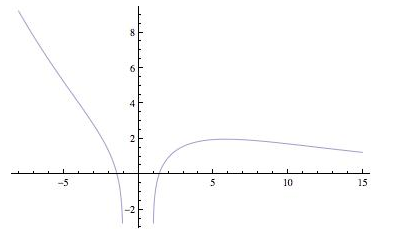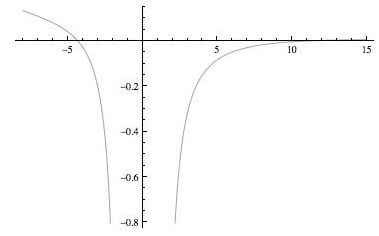# The x coordinate of the maximum and minimum points.### Single Variable Calculus: Concepts...

4th Edition
James Stewart
Publisher: Cengage Learning
ISBN: 9781337687805### Single Variable Calculus: Concepts...

4th Edition
James Stewart
Publisher: Cengage Learning
ISBN: 9781337687805

#### Solutions

Chapter 4, Problem 22RE
To determine

## To find: The x coordinate of the maximum and minimum points.

Expert Solution

The x coordinate of the minimum points are x=6 .

### Explanation of Solution

Given information:

The function is f(x)=e0.1xln(x21) .

Calculation:

Consider the equation.

f(x)=e0.1xln(x21)

The graph f(x) is shown figure-1Figure-1

The graph f(x) is shown figure-2Figure-2

The graph f(x) is shown figure-3Figure-3

The function has minimum value at x=6 and the inflection points for f(x) are x=4,11.5

### Have a homework question?

Subscribe to bartleby learn! Ask subject matter experts 30 homework questions each month. Plus, you’ll have access to millions of step-by-step textbook answers!